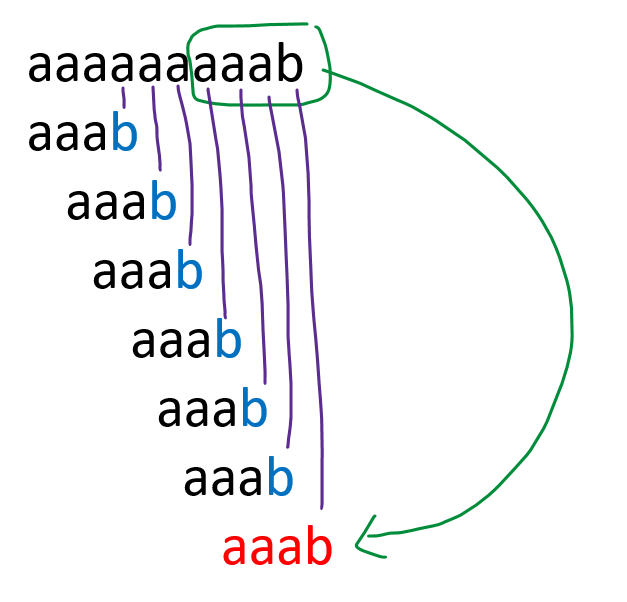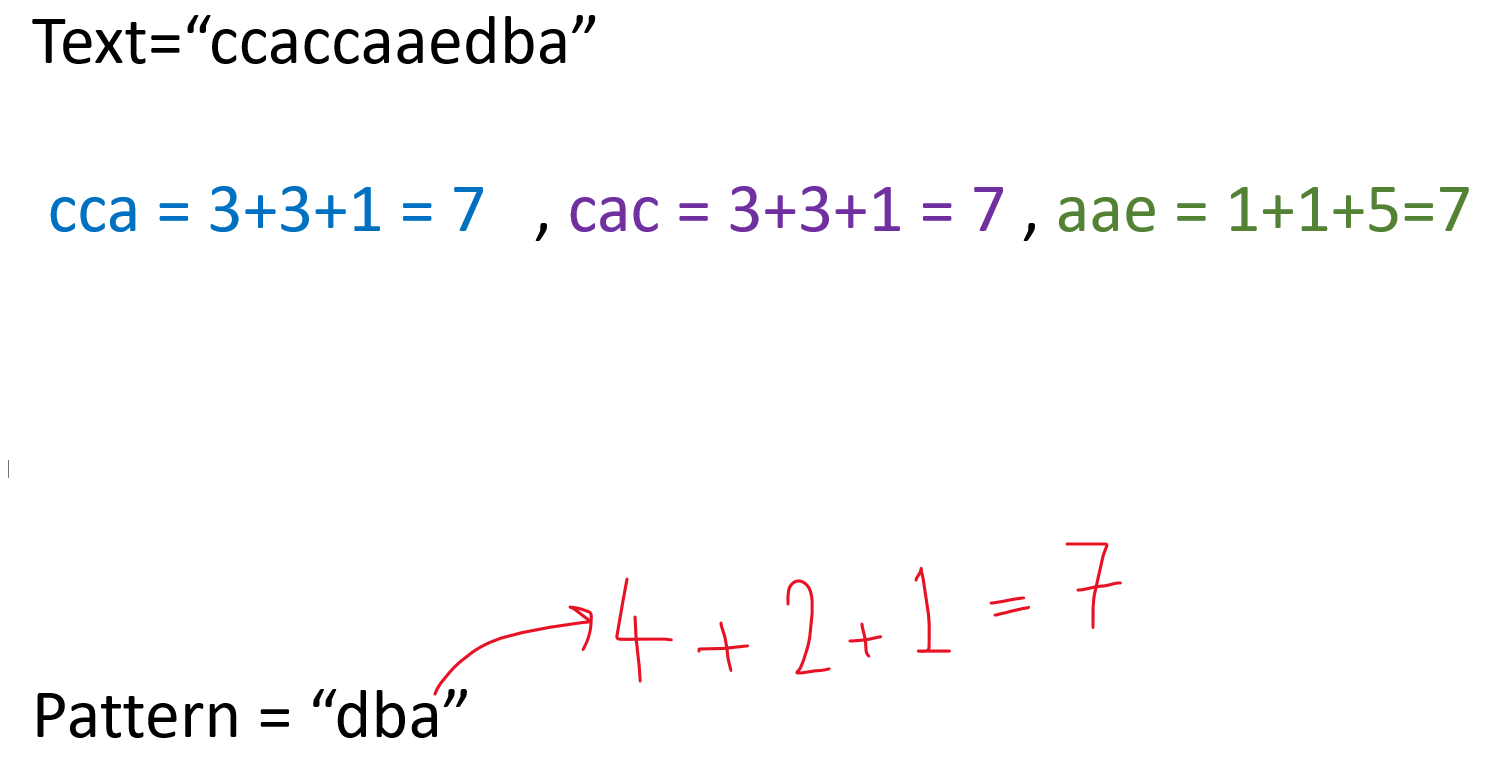# Rabin Karp Algorithm – Pattern Searching

Problem: Given a text and a pattern, write a function that prints all the occurrences of the pattern in the text. Assume the length of text  > pattern and both are given in the form of strings.

Examples:

```Example 1:
Input: text = “abccddaefg” , pattern=”cdd”
Output: 2
Explanation: “cdd” pattern is found at index 2.

Example 2:
Input: text="this is a test text" , pattern=”test”
Output: 10
Explanation: “test” pattern is found at index 10.
```

DisclaimerDon’t jump directly to the solution, try it out yourself first.

### Solution 1:

Intuition:

• We will use 2 nested loops, the outer loop will be used to iterate in a text string and the inner loop will be used to iterate in the pattern string.
• If we find a match, we will print the index and move on to find further patterns if they are present in the text or not.

Approach – Naive Approach

• We will slide the pattern string over the text string one by one. If we get a match, we will print the index and slide by 1 to check the remaining string.
• Let’s understand this with an example, suppose text=”aaaaaaaaab”, and pattern=”aaab”. Then this is how the algorithm will work.Code:

## C++ Code

``````#include <bits/stdc++.h>

using namespace std;

class Solution {
public:
void searchPattern(string & text, string & pattern) {

int n = text.length();
int m = pattern.length();

int j;
for (int i = 0; i <= n - m; i++) {

for (j = 0; j < m; ++j) {
if (pattern[j] != text[i + j]) {
break;
}
}

if (j == m) {
cout << "index = " << i << "\n";
}
}
}
};
int main() {

string text = "AABAACAADAABAABA";
string pattern = "AABA";

Solution obj;
obj.searchPattern(text, pattern);
return 0;
}``````

Output:

index = 0
index = 9
index = 12

Time complexity: O(m*(n-m+1)) [Worst case time complexity] where m = length of pattern, n = length of text.

Space complexity: O(1)

## Java Code

``````import java.util.*;

class TUF {

static void searchPattern(String text, String pattern) {

int n = text.length();
int m = pattern.length();

int j;
for (int i = 0; i <= n - m; i++) {

for (j = 0; j < m; ++j) {
if (pattern.charAt(j) != text.charAt(i + j)) {
break;
}
}

if (j == m) {
System.out.println("index = " + i);
}
}
}
public static void main(String args[]) {

String text = "AABAACAADAABAABA";
String pattern = "AABA";

searchPattern(text, pattern);
}
}``````

Output:

index = 0
index = 9
index = 12

Time complexity: O(m*(n-m+1)) [Worst case time complexity] where m = length of pattern, n = length of text.

Space complexity: O(1)

### Rabin Karp Algorithm

Before starting, let’s understand the hash function.

• Hash Function: It’s a function that is used to map data to fixed values. Eg: A function that converts a big phone number to a small practical integer value. This function maps a big number or string to a small integer that can be used as the index in the hash table.

So, let’s define a Hash Function for our algorithm. Let’s denote each letter of the alphabet with a code like, a is represented by 1, b is represented by 2, c – 3, d-4, ….z=26.

Let’s take an example of how this will help us.

`Text = “aaaaab”,Pattern= “aab”`
• Now as mentioned in the image, aab is represented by an integer which is 4 . We also got a substring in the Text string whose integer value is also 4. This value is called Hashcode.
• After getting the same hashcode, we need to cross-check whether both the strings are equal or not. In the above case, it’s equal.
• Now, this method has a DRAWBACK. After this, we will learn the actual algorithm.
• Let’s take an example, text=”ccaccaaedba”, pattern=”dba”As we can see the hashcodes of a substring of text are matching with the hashcode of the pattern but both strings are not the same. In this way, we are comparing patterns every time and it is not matching every time ( except “dba” in the above case)

• If we take such a simple Hash Function, there’s a possibility of collision with the other substrings which are having the same code though they are not patterns. This is also called Spurious Hits.
• In such a situation, the maximum time taken by the algorithm may be O(m*n).
• We selected such a type of hash function which is causing such Spurious Hits. So we need to change the Hash function.
• Now from here, we’ll be learning the actual algorithm.
```Hash Function = (pattern*(x^m-1) + pattern*(x^m-2) + pattern*(x^m-2) + ….pattern[m-1]*(x^0)) % MOD.

Here, x = base value ( number of alphabets used, if 26 alphabets are used in the text , then x=26 )
m = length of pattern.
```
• Inorder to minimize spurious hits, we use modulus.

Code:

## C++ Code

``````#include <bits/stdc++.h>

using namespace std;

void rabinKarpAlgo(string & pattern, string & text, int q) {

int m = pattern.size();
int n = text.size();
int i, j, hash_p = 0, hash_t = 0, h = 1, d = m + n;

for (i = 0; i < m - 1; i++) {
h = (h * d) % q;
}

// Calculate hash value for pattern and text
for (i = 0; i < m; i++) {
hash_p = (pattern[i] + d * hash_p) % q;
hash_t = (text[i] + d * hash_t) % q;
}

// Find the match
for (i = 0; i <= n - m; i++) {

// Check the hash values of current windows of text and pattern.
if (hash_p == hash_t) {

for (j = 0; j < m; j++) {

if (text[i + j] != pattern[j]) break;
}

// if pattern == text
if (j == m) cout << "Pattern is found at index : " << i << "\n";
}

// Calculate the hash value for next window : adding trailing digit
//and remove leading digit
if (i < n - m) {
hash_t = (d * (hash_t - text[i] * h) + text[i + m]) % q;

//converting negative value of hash_t to positive.
if (hash_t < 0) hash_t = (hash_t + q);
}
}
}

int main() {
string text = "GEEKS FOR GEEKS";
string pattern = "GEEK";
int q = 101;
rabinKarpAlgo(pattern, text, q);
}``````

Output:

Pattern is found at index: 0
Pattern is found at index: 10

Time complexity: O(n+m) [Average and best time complexity] , O(n*m) [worst complexity]

Worst case complexity occurs when all the characters of the pattern are the same as the text.

Space complexity: O(1)

``````import java.util.*;

class TUF {
static void rabinKarpAlgo(String pattern, String text, int q) {

int m = pattern.length();
int n = text.length();
int i, j, hash_p = 0, hash_t = 0, h = 1, d = m + n;

for (i = 0; i < m - 1; i++) {
h = (h * d) % q;
}

// Calculate hash value for pattern and text
for (i = 0; i < m; i++) {
hash_p = (pattern.charAt(i) + d * hash_p) % q;
hash_t = (text.charAt(i) + d * hash_t) % q;
}

// Find the match
for (i = 0; i <= n - m; i++) {

// Check the hash values of current windows of text and pattern.
if (hash_p == hash_t) {

for (j = 0; j < m; j++) {

if (text.charAt(i + j) != pattern.charAt(j)) break;
}

// if pattern == text
if (j == m) System.out.println("Pattern is found at index : " + i);
}

// Calculate the hash value for next window : adding trailing digit and
if (i < n - m) {
hash_t = (d * (hash_t - text.charAt(i) * h) + text.charAt(i + m)) % q;

//converting negative value of hash_t to positive.
if (hash_t < 0) hash_t = (hash_t + q);
}
}
}

public static void main(String args[]) {
String text = "GEEKS FOR GEEKS";
String pattern = "GEEK";
int q = 101;
rabinKarpAlgo(pattern, text, q);
}
}``````

Output:

Pattern is found at index: 0
Pattern is found at index: 10

Time complexity: O(n+m) [Average and best time complexity] , O(n*m) [worst complexity]

Worst case complexity occurs when all the characters of the pattern are the same as the text.

Space complexity: O(1)

Special thanks to Shreyas Vishwakarma for contributing to this article on takeUforward. If you also wish to share your knowledge with the takeUforward fam, please check out this article